# esm14722 operation and control of electric power systems

## Transcription

esm14722 operation and control of electric power systems
```ESM14722 OPERATION AND
CONTROL OF ELECTRIC
POWER SYSTEMS
Assoc.Prof.Dr. Bahtiyar DURSUN
Kırklareli University
2015,Kırklareli
The Economic Dispatch Problem
• Consider a system that consists of N
thermal-generating units serving an
aggregated electrical load, Pload
• input to each unit: cost rate of fuel
consumed, Fi
• output of each unit: electrical power
generated, Pi
• total cost rate, FT, is the sum of the
individual unit costs
• essential constraint: the sum of the output
powers must equal the load demand
• the problem is to minimize FT
2015, Kırklareli University
ESM14722 OPERATION AND CONTROL OF ELECTRIC POWER SYSTEMS
The Economic Dispatch Problem
• The mathematical statement of the problem is a constrained optimization with the following functions:
• objective function:
• equality constraint:
• note that any transmission losses are neglected and any operating limits are not explicitly stated when formulating this problem
• Problem may be solved using the Lagrange function
2015, Kırklareli University
ESM14722 OPERATION AND CONTROL OF ELECTRIC POWER SYSTEMS
The Economic Dispatch Problem
Principles
• the Lagrange function establishes the necessary conditions for finding an extrema
of an objective function with constraints
• taking the first derivatives of the Lagrange function with respect to the independent variables allows us to find the extreme value when the derivatives are set to zero
• there are NF + Nl derivatives, one for each independent variable and one for each equality constraint
• the derivatives of the Lagrange function with respect to the Lagrange multiplier l merely gives back the constraint equation the NF partial derivatives result in
2015, Kırklareli University
ESM14722 OPERATION AND CONTROL OF ELECTRIC POWER SYSTEMS
Example
• Determine the economic operating point for the three generating units when delivering a total of 850 MW
2015, Kırklareli University
ESM14722 OPERATION AND CONTROL OF ELECTRIC POWER SYSTEMS
Example
2015, Kırklareli University
ESM14722 OPERATION AND CONTROL OF ELECTRIC POWER SYSTEMS
The Economic Dispatch Problem
2015, Kırklareli University
ESM14722 OPERATION AND CONTROL OF ELECTRIC POWER SYSTEMS
Example
2015, Kırklareli University
ESM14722 OPERATION AND CONTROL OF ELECTRIC POWER SYSTEMS
Example
2015, Kırklareli University
ESM14722 OPERATION AND CONTROL OF ELECTRIC POWER SYSTEMS
Example
2015, Kırklareli University
ESM14722 OPERATION AND CONTROL OF ELECTRIC POWER SYSTEMS
Network Losses
• Consider a similar system, which now has a transmission network that connects the generating units to the load
• the economic dispatch problem is slightly more complicated
• the constraint equation must include the network losses, Ploss
• the objective function, FT is the same as before
• the constraint equation must be expanded as:
2015, Kırklareli University
ESM14722 OPERATION AND CONTROL OF ELECTRIC POWER SYSTEMS
Network Losses
2015, Kırklareli University
ESM14722 OPERATION AND CONTROL OF ELECTRIC POWER SYSTEMS
Network Losses
2015, Kırklareli University
ESM14722 OPERATION AND CONTROL OF ELECTRIC POWER SYSTEMS
Network Losses
2015, Kırklareli University
ESM14722 OPERATION AND CONTROL OF ELECTRIC POWER SYSTEMS
Network Losses
2015, Kırklareli University
ESM14722 OPERATION AND CONTROL OF ELECTRIC POWER SYSTEMS
Network Losses
2015, Kırklareli University
ESM14722 OPERATION AND CONTROL OF ELECTRIC POWER SYSTEMS
Network Losses
2015, Kırklareli University
ESM14722 OPERATION AND CONTROL OF ELECTRIC POWER SYSTEMS
```

### this PDF file - GAZI UNIVERSITY JOURNAL OF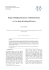More information

### Math 112 REVIEW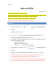More information

### ICS 271 Fall 2014 Instructor : Kalev Kask Homework Assignment 4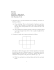More information

### Paşabahçe - Superbrands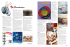More information

### MoSEFF Case Study - Covoare Ungheni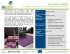More information

### First Derivatives Global Job Opportunities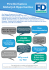More information

### When an emergency happens and lives are at stake, dispatchers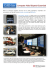More information

### Summer, 10 - LaGrange County Habitat for Humanity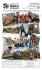More information

### LaGrange Friends Fall 2014 Newsletter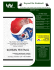More information